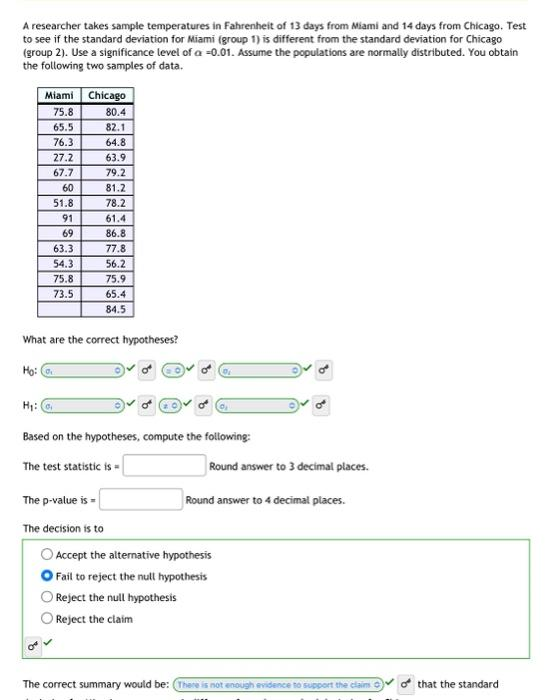Home / Expert Answers / Statistics and Probability / what-is-the-p-value-and-test-statistic-a-researcher-takes-sample-temperatures-in-fahrenheit-of-13-pa609

# (Solved): What is the P-Value and Test Statistic? A researcher takes sample temperatures in Fahrenheit of 13 ...

What is the P-Value and Test Statistic?A researcher takes sample temperatures in Fahrenheit of 13 days from Miami and 14 days from Chicago. Test. to see if the standard deviation for Miami (group 1) is different from the standard deviation for Chicago (group 2). Use a significance level of . Assume the populations are normally distributed. You obtain the following two samples of data. What are the correct hypotheses? Based on the hypotheses, compute the following: The test statistic is = Round answer to 3 decimal places. The -value is = Round answer to 4 decimal places. The decision is to Accept the alternative hypothesis Fail to reject the nult hypothesis Reject the null hypothesis Reject the claim

We have an Answer from Expert